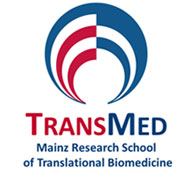﻿# Essential Statistics

Course description

The course will start out with descriptive statistics, including correlation, regression, and effect size measures. Touching upon probability theory, the course will then delve into the realms of inferential statistics. Rather than covering two dozen different hypothesis tests, the fact that all these tests follow a common logic ("null-hypothesis significance testing (NHST)") will be exploited. This logic can best be outlined using the binomial test, which is the test most amenable to students with limited knowledge of probability theory. Building on this foundation, the course explains the usage and pitfalls of some of the most common forms of NHST (z-test, t-test, analysis of variance, chi square test, nonparametric tests), with a special emphasis on statistical power. In addition, students are encouraged to approach their data with an exploratory attitude as outlined in Tukey’s seminal work on Exploratory Data Analysis. This is important because hypothesis tests yield highly processed data (p-values) that are prone to misinterpretation.

Target audience, number of participants

Doctoral candidates
Maximum number of participants: 20

Topics

• descriptive statistics, including correlation, regression and effect size measures
• binomial test
• usage and pitfalls of some of the most common forms of NHST (z-test, t-test, analysis of variance, chi square test, nonparametric tests)

Learning results

The teaching goal of this course is to provide a deeper understanding of statistical methods, which can be achieved by teaching students some of the basics of probability theory.

Preparation

Students are expected to bring their own laptops running copies of either Microsoft Excel or Open Office Calc, which will be used for some exercises.

The next workshop: to be announced.